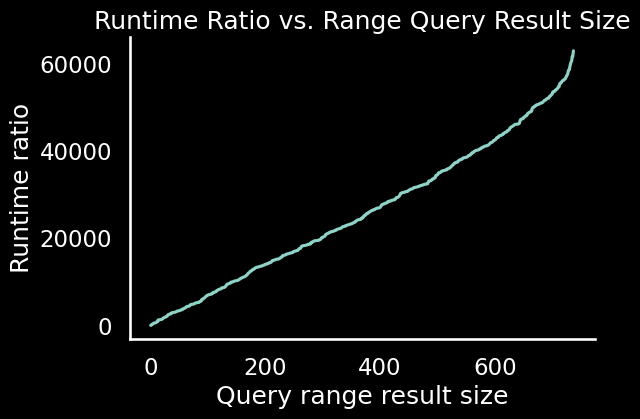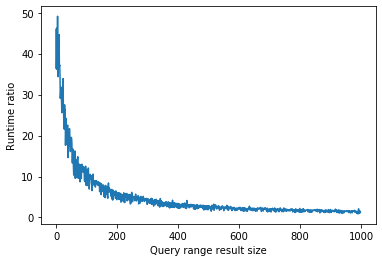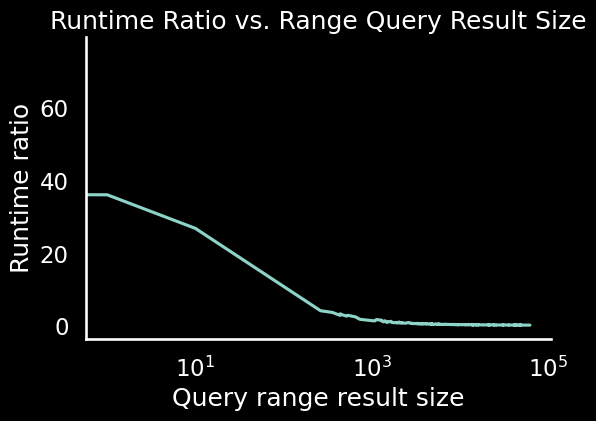# Is this a valid performance test? How does it compare to the solution notebook's test?

Guided project:
https://app.dataquest.io/c/109/m/530/guided-project%3A-implementing-a-key-value-database/12/performance-testing
Solution notebook:

I tried generating my own data for the key-value store performance test (compared to a dictionary `range_query` using `DictKVStore()`) at the end of the guided project.

To do this, I randomly generated 100,000 entries and 1,000 queries, resulting in roughly 730 result sizes with a range from 0 to ~730. After loading up the entries into each data storesorted and plotted the results

``````import pandas as pd
import random
import time
import csv
import matplotlib.pyplot as plt
import seaborn as sns

# Display graphs in notebook
%matplotlib inline

# Format graphs
sns.set(style='white', context='talk')
plt.style.use('dark_background')

# Generate 100,000 entries and 1,000 queries
random.seed(0)
key_values = [[random.randint(1, 100000), random.randint(1, 100000)] for _ in range(100000)]

range_start = [random.randint(1, 100000) for _ in range(1000)]

range_end = [random.randint(random.sample(range_start, 1), 100000) for _ in range(1000)]

range_queries = [(start, end) for start, end in zip(range_start, range_end)]

# Initialize data store classes
dict_kv = DictKVStore()
kvstore = KVStore()

# Load entries into each data structure
for key, value in key_values:
dict_kv[key] = value
kvstore[key] = value

# Measure query times
time_ratios_result_size = {}
for range_start, range_end in range_queries:

start = time.time()
result_size = len(dict_kv.range_query(range_start, range_end))
end = time.time()
time_dict = end - start

start = time.time()
result_size = len(kvstore.range_query(range_start, range_end))
end = time.time()
time_kv = end - start

time_ratios_result_size[result_size] = time_dict / time_kv

# Plot results
plt.plot(sorted(time_ratios_result_size))
plt.title('Runtime Ratio vs. Range Query Result Size')
plt.xlabel('Query range result size')
plt.ylabel('Runtime ratio')
sns.despine()
plt.show();
``````

Output:The solution notebook’s result (50,000 entries, 1,000 queries):My results suggest that my `KVStore` range query performs tens of thousands times better than the dictionary (`DictKVStore`) range query as the number or results for range queries increase.

Meanwhile the solution notebook’s results suggests the opposite. When result size increases, performance of the KVStore` goes down.

What’s going on here? Why am I seeing different trends?

So, full disclosure - I have no idea or knowledge about this particular content so I can’t jump deep into what exactly you are trying to do and I could be mistaken.

However, the important point here is to first look at your plot’s y-axis.

If you are plotting the runtime ratio, then it shouldn’t go that high. So, that’s the first indication something might be wrong.

Based on that, you should look at what you are trying to plot -

I can probably see your logic here - you want to plot your dictionary that’s been sorted based on the keys. So, what you think you are doing is your x-axis would be those sorted keys, and your y axis would be the runtime ratio values. Right?

The issue is that

``````sorted(dictitonary)
``````

results in a list. And this can be the confusing part. If you run the following -

``````type(sorted(time_ratios_result_size))
``````

it will return - `list`. If you try to `print` some values from the above -

``````print(sorted(time_ratios_result_size)[:10])
``````

You will get -

[0, 36, 230, 269, 313, 482, 517, 556, 578, 607]

And those are the keys of your dictionary. There are no runtime ratio values when you try to plot them. That’s why you sort of get that plot.

So, you need to figure out what keys and values your dictionary should be storing (because the solution code doesn’t use a dictionary) first, and then you need to extract those into their corresponding lists and try to plot those as needed.

For example, something like -

``````a = time_ratios_result_size

x = list(a.keys())
y = list(a.values())
``````

And then plot those as

``````plt.plot(x, y)
``````

or

``````plt.plot(sorted(x), y)
``````

depending on what you need.

1 Like

Thanks for taking a look doc. I see it was a simple mistake on my part.

I fixed the mistake with the following

``````# Plot results
sorted_results = sorted(time_ratios_result_size.items())
plt.semilogx([x for x, y in sorted_results], [y for x, y in sorted_results])
plt.title('Runtime Ratio vs. Range Query Result Size')
plt.xlabel('Query range result size')
plt.ylabel('Runtime ratio')
sns.despine()
plt.show();
``````

Output:1 Like